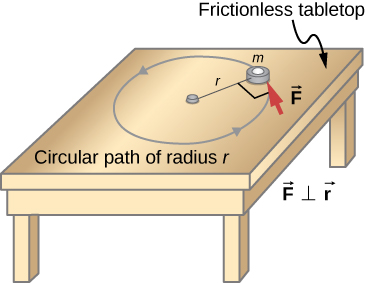# 10.7 Newton’s second law for rotation

 Page 1 / 5
By the end of this section, you will be able to:
• Calculate the torques on rotating systems about a fixed axis to find the angular acceleration
• Explain how changes in the moment of inertia of a rotating system affect angular acceleration with a fixed applied torque

In this section, we put together all the pieces learned so far in this chapter to analyze the dynamics of rotating rigid bodies. We have analyzed motion with kinematics and rotational kinetic energy but have not yet connected these ideas with force and/or torque. In this section, we introduce the rotational equivalent to Newton’s second law of motion and apply it to rigid bodies with fixed-axis rotation.

## Newton’s second law for rotation

We have thus far found many counterparts to the translational terms used throughout this text, most recently, torque, the rotational analog to force. This raises the question: Is there an analogous equation to Newton’s second law, $\Sigma \stackrel{\to }{F}=m\stackrel{\to }{a},$ which involves torque and rotational motion? To investigate this, we start with Newton’s second law for a single particle rotating around an axis and executing circular motion. Let’s exert a force $\stackrel{\to }{F}$ on a point mass m that is at a distance r from a pivot point ( [link] ). The particle is constrained to move in a circular path with fixed radius and the force is tangent to the circle. We apply Newton’s second law to determine the magnitude of the acceleration $a=F\text{/}m$ in the direction of $\stackrel{\to }{F}$ . Recall that the magnitude of the tangential acceleration is proportional to the magnitude of the angular acceleration by $a=r\alpha$ . Substituting this expression into Newton’s second law, we obtain

$F=mr\alpha .$An object is supported by a horizontal frictionless table and is attached to a pivot point by a cord that supplies centripetal force. A force F → is applied to the object perpendicular to the radius r , causing it to accelerate about the pivot point. The force is perpendicular to r .

Multiply both sides of this equation by r ,

$rF=m{r}^{2}\alpha .$

Note that the left side of this equation is the torque about the axis of rotation, where r is the lever arm and F is the force, perpendicular to r . Recall that the moment of inertia for a point particle is $I=m{r}^{2}$ . The torque applied perpendicularly to the point mass in [link] is therefore

$\tau =I\alpha .$

The torque on the particle is equal to the moment of inertia about the rotation axis times the angular acceleration . We can generalize this equation to a rigid body rotating about a fixed axis.

## Newton’s second law for rotation

If more than one torque acts on a rigid body about a fixed axis, then the sum of the torques equals the moment of inertia times the angular acceleration:

$\sum _{i}{\tau }_{i}=I\alpha .$

The term $I\alpha$ is a scalar quantity and can be positive or negative (counterclockwise or clockwise) depending upon the sign of the net torque. Remember the convention that counterclockwise angular acceleration is positive. Thus, if a rigid body is rotating clockwise and experiences a positive torque (counterclockwise), the angular acceleration is positive.

[link] is Newton’s second law for rotation    and tells us how to relate torque, moment of inertia, and rotational kinematics. This is called the equation for rotational dynamics    . With this equation, we can solve a whole class of problems involving force and rotation. It makes sense that the relationship for how much force it takes to rotate a body would include the moment of inertia, since that is the quantity that tells us how easy or hard it is to change the rotational motion of an object.

a length of copper wire was measured to be 50m with an uncertainty of 1cm, the thickness of the wire was measured to be 1mm with an uncertainty of 0.01mm, using a micrometer screw gauge, calculate the of copper wire used
If centripetal force is directed towards the center,why do you feel that you're thrown away from the center as a car goes around a curve? Explain
Which kind of wave does wind form
calculate the distance you will travel if you mantain an average speed of 10N m/s for 40 second
hw to calculate the momentum of the 2000.0 elephant change hunter at a speed of 7.50 m/s
how many cm makes 1 inches
2.5
omwoyo
2.54cm=1inche
omwoyo
how do we convert from m/s to km/hr
When paddling a canoe upstream, it is wisest to travel as near to the shore as possible. When canoeing downstream, it may be best to stay near the middle. Explain why?
Explain why polarization does not occur in sound
one ship sailing east with a speed of 7.5m/s passes a certain point at 8am and a second ship sailing north at the same speed passed the same point at 9.30am at what distance are they closet together and what is the distance between them then
density of a subtance is given as 360g/cm,put it in it s.i unit form
if m2 is twice of m1. find the ration of kinetic energy in COM system to lab system of elastic collision
What is a volt equal to?
list and explain the 3 ways of charging a conductor
conduction convention rubbing
Asdesaw
formula of magnetic field
why polarization does not occur in sound
Integral of a vector
define surface integral of a vector?
Rahat

#### Get Jobilize Job Search Mobile App in your pocket Now!By OpenStaxBy Lakeima RobertsBy Marion CabalfinBy OpenStaxBy OpenStaxBy OpenStaxBy OpenStaxBy Brooke DelaneyBy Robert MorrisBy Katie Montrose